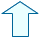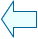LOGARITHMIC EQUATIONS AND SIMULTANEOUS LOGARITHMIC EQUATIONS Section : Algebra

1. EXPLANATION

Logarithmic equations are equations where the unknown(s) appear in the logarithm. For example: log(x+6) = 1 + log(x-3)

"Before continuing with this section you may find it useful to review the unit on logarithmic functions".

The type of logarithm which usually appears in logarithmic equations is a decimal or Naperian. The same base number is usually used throughout the equation.

They are all solved in the same way regardless of what the base number of the logarithm is. Therefore, in this section, unless indicated otherwise, we will refer to a logarithm as a log, which we understand to be in base 10.

However, remember that in the graphs in the windows, the program uses "log" to refer to the Naperian logarithms and "log10" to refer to the decimal ones. We will therefore use log10 for the graphical solutions in the exercises given.

Exercise 1.- Copy down the method used to solve the equation log(x+6) = log(2x-1) in your exercise book.

It seems logical that in order to satisfy this equation: x + 6 = 2x - 1 which means x = 7.

We have now solved the first logarithmic equation. Although the example in this case was very straight forward, we have seen the method which can be used to solve any logarithmic equation. We shall be explaining this method below.

 In order to solve these equations numerically we can divide them into two basic types, as indicated below:

 2.1. GRAPHICAL SOLUTIONS. EXAMPLE 1 Now we are going to see the graphical solution of the equation. As with all equations which have an unknown, there are two methods which can be used: 1.- Here is the first method. Make the equation equal to 0 and write the equation (which is a function) into the program: y =....LHS..... The solutions are the x values for the points where the graphs cut X-axis.  2.- Here is the second method. Draw the corresponding function graphs for each side of the equation and the values of x at the points where the two graphs intersect give us the solutions.  3.- Use the first method to find the solution of the equation and note that the process would be: log(x+6) - log(2x-1) = 0, then draw y = log(x+6) - log(2x-1). "The solution of the equation is the value of "x" at the point where the graph cuts the X-axis." You should soon find that x = 7. 4.- In the window, look at the straight line that cuts the X-axis at the same point (where x = 7). This is the graph of the equation x + 6 = 2x - 1, i.e.:  y = x+6 - (2x-1), which confirms that the method is accurate. This graphical method can be used to solve any logarithmic equation.

 2.2  GRAPHICAL SOLUTIONS. EXAMPLE 2 In the window below write the corresponding equations in the boxes to solve the following logarithmic equation, both with or without the use of logarithms. log(x2+2x) = log(3) (You should find two solutions).

3. NUMERICAL SOLUTIONS (IN GENERAL)

The method used to solve logarithmic equations numerically is based on the example in exercise 1. We need to therefore obtain a log(...) = log(...) type equation. In order to do so we must have a clear understanding of the following rules of logarithms:

 From the definition of a logarithm of number (a) in a certain base (b): logb(a)=n so that bn=a., we can deduce the rules of logarithms. log A + log B = log (A·B) (allows us to group logarithms added together in a single term)   log A - log B = log(A/B) (allows us to group logarithms subtracted from each other in a single term) n·log A = log An. (Used if necessary before the two rules above are applied). In this example remember that if "n" is a fraction then there will be a root in the logarithm.   n = log 10n (in particular: "0 = log 1"; 1 = log 10 ) These rules are usually used to solve most kinds of logarithmic equations.

1.- Solve the equation from the top of this page  log(x+6) = 1 + log(x-3) in your exercise book.

2.- Find the graphical solution for this equation in the window by changing the equation in the relevant box.

 Use either the first or second method explained above to solve the equation.

3.- If you didn't manage to solve the equation read the following steps carefully in order to do so:

log(x+6) = 1 + log(x-3) ; log(x+6) = log 10+ log(x-3) ; log(x+6) = log 10(x-3).

The equation is now simplified appropriately and the solution is the same as for the equation: x+6 = 10(x-3) which is x = 4.

4.- Solve the following equation in your exercise book: log 2 + log (11 - x2) = 2 log(5-x)

(You should end up with the quadratic equation: 3x2-10x+3 = 0).

(Use the window to solve the equation graphically).

 4.-THE VALIDITY OF THE SOLUTIONS In some cases, some of the solutions obtained of logarithmic equations may not be valid. Let's see why: 1.- Solve the equation log (3 - x2) =log 2 + log x 2.- Draw the graph of the equation y = log (3 - x2) - log 2 - log x in the following window. What do you notice about it? What are the solutions of the equation? 3.- Look carefully at the blue graph (a logarithmic equation) and note that it only cuts the X-axis at one point (x = 1 is a solution). However, the equation from log (3 - x2) =log 2x, is: 3 - x2 =2x, and whose graph is  y = 3 - x2 -2x  has two solutions. One solution is the one we already have x = 1 and the other is x = -3. But, why didn't the logarithmic equation give is the solution x = -3? 4.- "Some logarithmic equations give numerical solutions which are not valid. We need to check the validity of the solutions we get by putting them back into the original equation". Obviously, if we use the graphical method we saw earlier, we don't have to check the validity of the solutions as the solutions found using this method are always valid. 5.- Solve the equations below numerically in your exercise book and check the validity of the solutions you obtain. Then, solve them graphically in the window above. a) 2log(x) - log ( x2 - 6) = 1 b) log (5x+4) - log 2 = (1/2) log(x+4)

 5.-solVING SIMULTANEOUS EQUATIONS Simultaneous logarithmic equations are essentially solved in the same way as other equations. Each equation is dealt with individually as explained above and then they are solved simultaneously (without logarithms). For example: 1.- Solve these simultaneous equations: x - y = 9 log x + log y = 1 Note that the first equation in this case does not include any logarithms and the second is simplified easily to x·y = 10, and If we solve the resulting simultaneous equations we get the solutions: x = 10 and y = 1. In order to find the graphical solution just write both equations in the boxes in the lower part of the window (in the form in which they are expressed) and the value of "x" at the point of intersection gives the solution. (In this case we do not draw the graphs of the algebraic equations (without logarithms) as too many graphs could be confusing. However, they would be drawn as we did before) "For more information about solving simultaneous equations graphically refer to the unit on "simultaneous equations". Note: Remember that when we draw graphs of equations which include two variables (x and y) with logarithms, we do not always get a clear curve. This is due to problems with the existence of a logarithmic function. You should also bear in mind that in some cases you may need to alter the scale accordingly. Most of the time you will need to reduce it!  Exercise 8.- Solve the following simultaneous equations numerically and check the solution graphically in the window above. log(x) - log(y) = 1 x + y = 22Leoncio Santos CuervoSpanish Ministry of Education. Year 2001